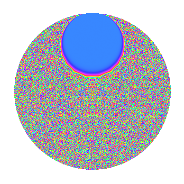Properties

 Label 2.50.a.bLevel 2 Weight 50 Character orbit 2.a Self dual Yes Analytic conductor 30.413 Analytic rank 0 Dimension 3 CM No Inner twists 1

Related objects

Newspace parameters

 Level: $$N$$ = $$2$$ Weight: $$k$$ = $$50$$ Character orbit: $$[\chi]$$ = 2.a (trivial)

Newform invariants

 Self dual: Yes Analytic conductor: $$30.4132410198$$ Analytic rank: $$0$$ Dimension: $$3$$ Coefficient field: $$\mathbb{Q}[x]/(x^{3} - \cdots)$$ Coefficient ring: $$\Z[a_1, \ldots, a_{5}]$$ Coefficient ring index: $$2^{22}\cdot 3^{5}\cdot 5^{2}\cdot 7^{2}$$ Fricke sign: $$-1$$ Sato-Tate group: $\mathrm{SU}(2)$

$q$-expansion

Coefficients of the $$q$$-expansion are expressed in terms of a basis $$1,\beta_1,\beta_2$$ for the coefficient ring described below. We also show the integral $$q$$-expansion of the trace form.

 $$f(q)$$ $$=$$ $$q$$ $$+ 16777216 q^{2}$$ $$+ ( -5401204796 - \beta_{1} ) q^{3}$$ $$+ 281474976710656 q^{4}$$ $$+ ( -33937672133946810 - 78841 \beta_{1} - \beta_{2} ) q^{5}$$ $$+ ( -90617179522727936 - 16777216 \beta_{1} ) q^{6}$$ $$+ ( -41826393076734599032 + 99835658 \beta_{1} - 3700 \beta_{2} ) q^{7}$$ $$+ 4722366482869645213696 q^{8}$$ $$+ ( 129810977173232307350733 - 175523252718 \beta_{1} + 1418850 \beta_{2} ) q^{9}$$ $$+O(q^{10})$$ $$q$$ $$+16777216 q^{2}$$ $$+(-5401204796 - \beta_{1}) q^{3}$$ $$+281474976710656 q^{4}$$ $$+(-33937672133946810 - 78841 \beta_{1} - \beta_{2}) q^{5}$$ $$+(-90617179522727936 - 16777216 \beta_{1}) q^{6}$$ $$+(-41826393076734599032 + 99835658 \beta_{1} - 3700 \beta_{2}) q^{7}$$ $$+$$$$47\!\cdots\!96$$$$q^{8}$$ $$+($$$$12\!\cdots\!33$$$$- 175523252718 \beta_{1} + 1418850 \beta_{2}) q^{9}$$ $$+(-$$$$56\!\cdots\!60$$$$- 1322732486656 \beta_{1} - 16777216 \beta_{2}) q^{10}$$ $$+(-$$$$86\!\cdots\!68$$$$- 76202146518283 \beta_{1} + 52537400 \beta_{2}) q^{11}$$ $$+(-$$$$15\!\cdots\!76$$$$- 281474976710656 \beta_{1}) q^{12}$$ $$+($$$$98\!\cdots\!14$$$$- 340286117859137 \beta_{1} + 4260419575 \beta_{2}) q^{13}$$ $$+(-$$$$70\!\cdots\!12$$$$+ 1674964398768128 \beta_{1} - 62075699200 \beta_{2}) q^{14}$$ $$+($$$$29\!\cdots\!60$$$$+ 125268300209547246 \beta_{1} + 303590419956 \beta_{2}) q^{15}$$ $$+$$$$79\!\cdots\!36$$$$q^{16}$$ $$+($$$$13\!\cdots\!18$$$$- 2043132990929384798 \beta_{1} - 6325836222350 \beta_{2}) q^{17}$$ $$+($$$$21\!\cdots\!28$$$$- 2944791523872473088 \beta_{1} + 23804352921600 \beta_{2}) q^{18}$$ $$+($$$$48\!\cdots\!60$$$$+ 18528996595190482627 \beta_{1} + 8505702406600 \beta_{2}) q^{19}$$ $$+(-$$$$95\!\cdots\!60$$$$- 22191768638844829696 \beta_{1} - 281474976710656 \beta_{2}) q^{20}$$ $$+(-$$$$36\!\cdots\!28$$$$+$$$$45\!\cdots\!44$$$$\beta_{1} + 567737584938900 \beta_{2}) q^{21}$$ $$+(-$$$$14\!\cdots\!88$$$$-$$$$12\!\cdots\!28$$$$\beta_{1} + 881431307878400 \beta_{2}) q^{22}$$ $$+(-$$$$16\!\cdots\!36$$$$-$$$$67\!\cdots\!66$$$$\beta_{1} - 4043926350742700 \beta_{2}) q^{23}$$ $$+(-$$$$25\!\cdots\!16$$$$-$$$$47\!\cdots\!96$$$$\beta_{1}) q^{24}$$ $$+($$$$13\!\cdots\!75$$$$+$$$$34\!\cdots\!80$$$$\beta_{1} + 480088492044180 \beta_{2}) q^{25}$$ $$+($$$$16\!\cdots\!24$$$$-$$$$57\!\cdots\!92$$$$\beta_{1} + 71477979460403200 \beta_{2}) q^{26}$$ $$+($$$$65\!\cdots\!00$$$$-$$$$72\!\cdots\!02$$$$\beta_{1} - 22990498274413800 \beta_{2}) q^{27}$$ $$+(-$$$$11\!\cdots\!92$$$$+$$$$28\!\cdots\!48$$$$\beta_{1} - 1041457413829427200 \beta_{2}) q^{28}$$ $$+($$$$31\!\cdots\!10$$$$-$$$$97\!\cdots\!81$$$$\beta_{1} + 2068317919687048475 \beta_{2}) q^{29}$$ $$+($$$$49\!\cdots\!60$$$$+$$$$21\!\cdots\!36$$$$\beta_{1} + 5093402051132522496 \beta_{2}) q^{30}$$ $$+($$$$80\!\cdots\!52$$$$+$$$$21\!\cdots\!04$$$$\beta_{1} - 21160996591207173600 \beta_{2}) q^{31}$$ $$+$$$$13\!\cdots\!76$$$$q^{32}$$ $$+($$$$28\!\cdots\!28$$$$-$$$$10\!\cdots\!94$$$$\beta_{1} + 98046584479571070150 \beta_{2}) q^{33}$$ $$+($$$$21\!\cdots\!88$$$$-$$$$34\!\cdots\!68$$$$\beta_{1} -$$$$10\!\cdots\!00$$$$\beta_{2}) q^{34}$$ $$+($$$$10\!\cdots\!20$$$$+$$$$72\!\cdots\!32$$$$\beta_{1} -$$$$19\!\cdots\!48$$$$\beta_{2}) q^{35}$$ $$+($$$$36\!\cdots\!48$$$$-$$$$49\!\cdots\!08$$$$\beta_{1} +$$$$39\!\cdots\!00$$$$\beta_{2}) q^{36}$$ $$+($$$$10\!\cdots\!58$$$$+$$$$22\!\cdots\!27$$$$\beta_{1} - 1016938682892827725 \beta_{2}) q^{37}$$ $$+($$$$81\!\cdots\!60$$$$+$$$$31\!\cdots\!32$$$$\beta_{1} +$$$$14\!\cdots\!00$$$$\beta_{2}) q^{38}$$ $$+($$$$12\!\cdots\!56$$$$-$$$$14\!\cdots\!82$$$$\beta_{1} -$$$$33\!\cdots\!00$$$$\beta_{2}) q^{39}$$ $$+(-$$$$16\!\cdots\!60$$$$-$$$$37\!\cdots\!36$$$$\beta_{1} -$$$$47\!\cdots\!96$$$$\beta_{2}) q^{40}$$ $$+(-$$$$38\!\cdots\!18$$$$-$$$$10\!\cdots\!04$$$$\beta_{1} +$$$$88\!\cdots\!00$$$$\beta_{2}) q^{41}$$ $$+(-$$$$61\!\cdots\!48$$$$+$$$$75\!\cdots\!04$$$$\beta_{1} +$$$$95\!\cdots\!00$$$$\beta_{2}) q^{42}$$ $$+(-$$$$58\!\cdots\!16$$$$+$$$$10\!\cdots\!57$$$$\beta_{1} -$$$$32\!\cdots\!00$$$$\beta_{2}) q^{43}$$ $$+(-$$$$24\!\cdots\!08$$$$-$$$$21\!\cdots\!48$$$$\beta_{1} +$$$$14\!\cdots\!00$$$$\beta_{2}) q^{44}$$ $$+(-$$$$38\!\cdots\!30$$$$-$$$$19\!\cdots\!73$$$$\beta_{1} +$$$$33\!\cdots\!47$$$$\beta_{2}) q^{45}$$ $$+(-$$$$27\!\cdots\!76$$$$-$$$$11\!\cdots\!56$$$$\beta_{1} -$$$$67\!\cdots\!00$$$$\beta_{2}) q^{46}$$ $$+(-$$$$72\!\cdots\!52$$$$+$$$$72\!\cdots\!88$$$$\beta_{1} +$$$$31\!\cdots\!00$$$$\beta_{2}) q^{47}$$ $$+(-$$$$42\!\cdots\!56$$$$-$$$$79\!\cdots\!36$$$$\beta_{1}) q^{48}$$ $$+($$$$12\!\cdots\!17$$$$+$$$$10\!\cdots\!48$$$$\beta_{1} -$$$$12\!\cdots\!00$$$$\beta_{2}) q^{49}$$ $$+($$$$22\!\cdots\!00$$$$+$$$$58\!\cdots\!80$$$$\beta_{1} +$$$$80\!\cdots\!80$$$$\beta_{2}) q^{50}$$ $$+($$$$74\!\cdots\!72$$$$-$$$$10\!\cdots\!90$$$$\beta_{1} +$$$$41\!\cdots\!00$$$$\beta_{2}) q^{51}$$ $$+($$$$27\!\cdots\!84$$$$-$$$$95\!\cdots\!72$$$$\beta_{1} +$$$$11\!\cdots\!00$$$$\beta_{2}) q^{52}$$ $$+($$$$24\!\cdots\!34$$$$-$$$$10\!\cdots\!37$$$$\beta_{1} -$$$$14\!\cdots\!25$$$$\beta_{2}) q^{53}$$ $$+($$$$10\!\cdots\!00$$$$-$$$$12\!\cdots\!32$$$$\beta_{1} -$$$$38\!\cdots\!00$$$$\beta_{2}) q^{54}$$ $$+($$$$10\!\cdots\!80$$$$+$$$$90\!\cdots\!18$$$$\beta_{1} +$$$$34\!\cdots\!48$$$$\beta_{2}) q^{55}$$ $$+(-$$$$19\!\cdots\!72$$$$+$$$$47\!\cdots\!68$$$$\beta_{1} -$$$$17\!\cdots\!00$$$$\beta_{2}) q^{56}$$ $$+(-$$$$68\!\cdots\!60$$$$-$$$$24\!\cdots\!82$$$$\beta_{1} -$$$$27\!\cdots\!50$$$$\beta_{2}) q^{57}$$ $$+($$$$52\!\cdots\!60$$$$-$$$$16\!\cdots\!96$$$$\beta_{1} +$$$$34\!\cdots\!00$$$$\beta_{2}) q^{58}$$ $$+(-$$$$17\!\cdots\!80$$$$-$$$$19\!\cdots\!47$$$$\beta_{1} -$$$$81\!\cdots\!00$$$$\beta_{2}) q^{59}$$ $$+($$$$82\!\cdots\!60$$$$+$$$$35\!\cdots\!76$$$$\beta_{1} +$$$$85\!\cdots\!36$$$$\beta_{2}) q^{60}$$ $$+(-$$$$41\!\cdots\!98$$$$+$$$$48\!\cdots\!43$$$$\beta_{1} +$$$$18\!\cdots\!75$$$$\beta_{2}) q^{61}$$ $$+($$$$13\!\cdots\!32$$$$+$$$$35\!\cdots\!64$$$$\beta_{1} -$$$$35\!\cdots\!00$$$$\beta_{2}) q^{62}$$ $$+(-$$$$15\!\cdots\!56$$$$+$$$$34\!\cdots\!30$$$$\beta_{1} +$$$$13\!\cdots\!00$$$$\beta_{2}) q^{63}$$ $$+$$$$22\!\cdots\!16$$$$q^{64}$$ $$+(-$$$$14\!\cdots\!40$$$$-$$$$12\!\cdots\!04$$$$\beta_{1} -$$$$63\!\cdots\!44$$$$\beta_{2}) q^{65}$$ $$+($$$$47\!\cdots\!48$$$$-$$$$17\!\cdots\!04$$$$\beta_{1} +$$$$16\!\cdots\!00$$$$\beta_{2}) q^{66}$$ $$+($$$$37\!\cdots\!08$$$$-$$$$51\!\cdots\!01$$$$\beta_{1} -$$$$27\!\cdots\!00$$$$\beta_{2}) q^{67}$$ $$+($$$$36\!\cdots\!08$$$$-$$$$57\!\cdots\!88$$$$\beta_{1} -$$$$17\!\cdots\!00$$$$\beta_{2}) q^{68}$$ $$+($$$$25\!\cdots\!56$$$$+$$$$19\!\cdots\!12$$$$\beta_{1} +$$$$17\!\cdots\!00$$$$\beta_{2}) q^{69}$$ $$+($$$$16\!\cdots\!20$$$$+$$$$12\!\cdots\!12$$$$\beta_{1} -$$$$33\!\cdots\!68$$$$\beta_{2}) q^{70}$$ $$+(-$$$$13\!\cdots\!08$$$$-$$$$18\!\cdots\!62$$$$\beta_{1} +$$$$31\!\cdots\!00$$$$\beta_{2}) q^{71}$$ $$+($$$$61\!\cdots\!68$$$$-$$$$82\!\cdots\!28$$$$\beta_{1} +$$$$67\!\cdots\!00$$$$\beta_{2}) q^{72}$$ $$+(-$$$$33\!\cdots\!06$$$$-$$$$40\!\cdots\!62$$$$\beta_{1} +$$$$60\!\cdots\!50$$$$\beta_{2}) q^{73}$$ $$+($$$$17\!\cdots\!28$$$$+$$$$36\!\cdots\!32$$$$\beta_{1} -$$$$17\!\cdots\!00$$$$\beta_{2}) q^{74}$$ $$+(-$$$$12\!\cdots\!00$$$$-$$$$69\!\cdots\!55$$$$\beta_{1} -$$$$49\!\cdots\!80$$$$\beta_{2}) q^{75}$$ $$+($$$$13\!\cdots\!60$$$$+$$$$52\!\cdots\!12$$$$\beta_{1} +$$$$23\!\cdots\!00$$$$\beta_{2}) q^{76}$$ $$+(-$$$$77\!\cdots\!24$$$$+$$$$31\!\cdots\!52$$$$\beta_{1} +$$$$92\!\cdots\!00$$$$\beta_{2}) q^{77}$$ $$+($$$$20\!\cdots\!96$$$$-$$$$25\!\cdots\!12$$$$\beta_{1} -$$$$56\!\cdots\!00$$$$\beta_{2}) q^{78}$$ $$+($$$$12\!\cdots\!20$$$$+$$$$32\!\cdots\!32$$$$\beta_{1} -$$$$91\!\cdots\!00$$$$\beta_{2}) q^{79}$$ $$+(-$$$$26\!\cdots\!60$$$$-$$$$62\!\cdots\!76$$$$\beta_{1} -$$$$79\!\cdots\!36$$$$\beta_{2}) q^{80}$$ $$+(-$$$$48\!\cdots\!39$$$$-$$$$33\!\cdots\!34$$$$\beta_{1} -$$$$23\!\cdots\!50$$$$\beta_{2}) q^{81}$$ $$+(-$$$$63\!\cdots\!88$$$$-$$$$17\!\cdots\!64$$$$\beta_{1} +$$$$14\!\cdots\!00$$$$\beta_{2}) q^{82}$$ $$+($$$$50\!\cdots\!64$$$$-$$$$72\!\cdots\!65$$$$\beta_{1} +$$$$57\!\cdots\!00$$$$\beta_{2}) q^{83}$$ $$+(-$$$$10\!\cdots\!68$$$$+$$$$12\!\cdots\!64$$$$\beta_{1} +$$$$15\!\cdots\!00$$$$\beta_{2}) q^{84}$$ $$+($$$$18\!\cdots\!20$$$$+$$$$29\!\cdots\!42$$$$\beta_{1} -$$$$10\!\cdots\!38$$$$\beta_{2}) q^{85}$$ $$+(-$$$$98\!\cdots\!56$$$$+$$$$17\!\cdots\!12$$$$\beta_{1} -$$$$54\!\cdots\!00$$$$\beta_{2}) q^{86}$$ $$+($$$$35\!\cdots\!40$$$$-$$$$70\!\cdots\!94$$$$\beta_{1} +$$$$98\!\cdots\!00$$$$\beta_{2}) q^{87}$$ $$+(-$$$$40\!\cdots\!28$$$$-$$$$35\!\cdots\!68$$$$\beta_{1} +$$$$24\!\cdots\!00$$$$\beta_{2}) q^{88}$$ $$+($$$$18\!\cdots\!90$$$$+$$$$60\!\cdots\!10$$$$\beta_{1} +$$$$75\!\cdots\!50$$$$\beta_{2}) q^{89}$$ $$+(-$$$$64\!\cdots\!80$$$$-$$$$33\!\cdots\!68$$$$\beta_{1} +$$$$56\!\cdots\!52$$$$\beta_{2}) q^{90}$$ $$+(-$$$$48\!\cdots\!48$$$$+$$$$77\!\cdots\!56$$$$\beta_{1} -$$$$18\!\cdots\!00$$$$\beta_{2}) q^{91}$$ $$+(-$$$$46\!\cdots\!16$$$$-$$$$18\!\cdots\!96$$$$\beta_{1} -$$$$11\!\cdots\!00$$$$\beta_{2}) q^{92}$$ $$+(-$$$$78\!\cdots\!92$$$$+$$$$18\!\cdots\!04$$$$\beta_{1} +$$$$10\!\cdots\!00$$$$\beta_{2}) q^{93}$$ $$+(-$$$$12\!\cdots\!32$$$$+$$$$12\!\cdots\!08$$$$\beta_{1} +$$$$52\!\cdots\!00$$$$\beta_{2}) q^{94}$$ $$+(-$$$$93\!\cdots\!00$$$$-$$$$28\!\cdots\!30$$$$\beta_{1} -$$$$99\!\cdots\!80$$$$\beta_{2}) q^{95}$$ $$+(-$$$$71\!\cdots\!96$$$$-$$$$13\!\cdots\!76$$$$\beta_{1}) q^{96}$$ $$+($$$$14\!\cdots\!38$$$$+$$$$28\!\cdots\!30$$$$\beta_{1} +$$$$28\!\cdots\!50$$$$\beta_{2}) q^{97}$$ $$+($$$$20\!\cdots\!72$$$$+$$$$17\!\cdots\!68$$$$\beta_{1} -$$$$21\!\cdots\!00$$$$\beta_{2}) q^{98}$$ $$+($$$$58\!\cdots\!56$$$$-$$$$22\!\cdots\!55$$$$\beta_{1} -$$$$16\!\cdots\!00$$$$\beta_{2}) q^{99}$$ $$+O(q^{100})$$ $$\operatorname{Tr}(f)(q)$$ $$=$$ $$3q$$ $$\mathstrut +\mathstrut 50331648q^{2}$$ $$\mathstrut -\mathstrut 16203614388q^{3}$$ $$\mathstrut +\mathstrut 844424930131968q^{4}$$ $$\mathstrut -\mathstrut 101813016401840430q^{5}$$ $$\mathstrut -\mathstrut 271851538568183808q^{6}$$ $$\mathstrut -\mathstrut 125479179230203797096q^{7}$$ $$\mathstrut +\mathstrut 14167099448608935641088q^{8}$$ $$\mathstrut +\mathstrut 389432931519696922052199q^{9}$$ $$\mathstrut +\mathstrut O(q^{10})$$ $$3q$$ $$\mathstrut +\mathstrut 50331648q^{2}$$ $$\mathstrut -\mathstrut 16203614388q^{3}$$ $$\mathstrut +\mathstrut 844424930131968q^{4}$$ $$\mathstrut -\mathstrut 101813016401840430q^{5}$$ $$\mathstrut -\mathstrut 271851538568183808q^{6}$$ $$\mathstrut -\mathstrut$$$$12\!\cdots\!96$$$$q^{7}$$ $$\mathstrut +\mathstrut$$$$14\!\cdots\!88$$$$q^{8}$$ $$\mathstrut +\mathstrut$$$$38\!\cdots\!99$$$$q^{9}$$ $$\mathstrut -\mathstrut$$$$17\!\cdots\!80$$$$q^{10}$$ $$\mathstrut -\mathstrut$$$$25\!\cdots\!04$$$$q^{11}$$ $$\mathstrut -\mathstrut$$$$45\!\cdots\!28$$$$q^{12}$$ $$\mathstrut +\mathstrut$$$$29\!\cdots\!42$$$$q^{13}$$ $$\mathstrut -\mathstrut$$$$21\!\cdots\!36$$$$q^{14}$$ $$\mathstrut +\mathstrut$$$$87\!\cdots\!80$$$$q^{15}$$ $$\mathstrut +\mathstrut$$$$23\!\cdots\!08$$$$q^{16}$$ $$\mathstrut +\mathstrut$$$$39\!\cdots\!54$$$$q^{17}$$ $$\mathstrut +\mathstrut$$$$65\!\cdots\!84$$$$q^{18}$$ $$\mathstrut +\mathstrut$$$$14\!\cdots\!80$$$$q^{19}$$ $$\mathstrut -\mathstrut$$$$28\!\cdots\!80$$$$q^{20}$$ $$\mathstrut -\mathstrut$$$$10\!\cdots\!84$$$$q^{21}$$ $$\mathstrut -\mathstrut$$$$43\!\cdots\!64$$$$q^{22}$$ $$\mathstrut -\mathstrut$$$$49\!\cdots\!08$$$$q^{23}$$ $$\mathstrut -\mathstrut$$$$76\!\cdots\!48$$$$q^{24}$$ $$\mathstrut +\mathstrut$$$$39\!\cdots\!25$$$$q^{25}$$ $$\mathstrut +\mathstrut$$$$49\!\cdots\!72$$$$q^{26}$$ $$\mathstrut +\mathstrut$$$$19\!\cdots\!00$$$$q^{27}$$ $$\mathstrut -\mathstrut$$$$35\!\cdots\!76$$$$q^{28}$$ $$\mathstrut +\mathstrut$$$$93\!\cdots\!30$$$$q^{29}$$ $$\mathstrut +\mathstrut$$$$14\!\cdots\!80$$$$q^{30}$$ $$\mathstrut +\mathstrut$$$$24\!\cdots\!56$$$$q^{31}$$ $$\mathstrut +\mathstrut$$$$39\!\cdots\!28$$$$q^{32}$$ $$\mathstrut +\mathstrut$$$$84\!\cdots\!84$$$$q^{33}$$ $$\mathstrut +\mathstrut$$$$65\!\cdots\!64$$$$q^{34}$$ $$\mathstrut +\mathstrut$$$$30\!\cdots\!60$$$$q^{35}$$ $$\mathstrut +\mathstrut$$$$10\!\cdots\!44$$$$q^{36}$$ $$\mathstrut +\mathstrut$$$$30\!\cdots\!74$$$$q^{37}$$ $$\mathstrut +\mathstrut$$$$24\!\cdots\!80$$$$q^{38}$$ $$\mathstrut +\mathstrut$$$$36\!\cdots\!68$$$$q^{39}$$ $$\mathstrut -\mathstrut$$$$48\!\cdots\!80$$$$q^{40}$$ $$\mathstrut -\mathstrut$$$$11\!\cdots\!54$$$$q^{41}$$ $$\mathstrut -\mathstrut$$$$18\!\cdots\!44$$$$q^{42}$$ $$\mathstrut -\mathstrut$$$$17\!\cdots\!48$$$$q^{43}$$ $$\mathstrut -\mathstrut$$$$72\!\cdots\!24$$$$q^{44}$$ $$\mathstrut -\mathstrut$$$$11\!\cdots\!90$$$$q^{45}$$ $$\mathstrut -\mathstrut$$$$83\!\cdots\!28$$$$q^{46}$$ $$\mathstrut -\mathstrut$$$$21\!\cdots\!56$$$$q^{47}$$ $$\mathstrut -\mathstrut$$$$12\!\cdots\!68$$$$q^{48}$$ $$\mathstrut +\mathstrut$$$$37\!\cdots\!51$$$$q^{49}$$ $$\mathstrut +\mathstrut$$$$66\!\cdots\!00$$$$q^{50}$$ $$\mathstrut +\mathstrut$$$$22\!\cdots\!16$$$$q^{51}$$ $$\mathstrut +\mathstrut$$$$82\!\cdots\!52$$$$q^{52}$$ $$\mathstrut +\mathstrut$$$$74\!\cdots\!02$$$$q^{53}$$ $$\mathstrut +\mathstrut$$$$32\!\cdots\!00$$$$q^{54}$$ $$\mathstrut +\mathstrut$$$$32\!\cdots\!40$$$$q^{55}$$ $$\mathstrut -\mathstrut$$$$59\!\cdots\!16$$$$q^{56}$$ $$\mathstrut -\mathstrut$$$$20\!\cdots\!80$$$$q^{57}$$ $$\mathstrut +\mathstrut$$$$15\!\cdots\!80$$$$q^{58}$$ $$\mathstrut -\mathstrut$$$$53\!\cdots\!40$$$$q^{59}$$ $$\mathstrut +\mathstrut$$$$24\!\cdots\!80$$$$q^{60}$$ $$\mathstrut -\mathstrut$$$$12\!\cdots\!94$$$$q^{61}$$ $$\mathstrut +\mathstrut$$$$40\!\cdots\!96$$$$q^{62}$$ $$\mathstrut -\mathstrut$$$$46\!\cdots\!68$$$$q^{63}$$ $$\mathstrut +\mathstrut$$$$66\!\cdots\!48$$$$q^{64}$$ $$\mathstrut -\mathstrut$$$$42\!\cdots\!20$$$$q^{65}$$ $$\mathstrut +\mathstrut$$$$14\!\cdots\!44$$$$q^{66}$$ $$\mathstrut +\mathstrut$$$$11\!\cdots\!24$$$$q^{67}$$ $$\mathstrut +\mathstrut$$$$11\!\cdots\!24$$$$q^{68}$$ $$\mathstrut +\mathstrut$$$$77\!\cdots\!68$$$$q^{69}$$ $$\mathstrut +\mathstrut$$$$50\!\cdots\!60$$$$q^{70}$$ $$\mathstrut -\mathstrut$$$$40\!\cdots\!24$$$$q^{71}$$ $$\mathstrut +\mathstrut$$$$18\!\cdots\!04$$$$q^{72}$$ $$\mathstrut -\mathstrut$$$$10\!\cdots\!18$$$$q^{73}$$ $$\mathstrut +\mathstrut$$$$51\!\cdots\!84$$$$q^{74}$$ $$\mathstrut -\mathstrut$$$$38\!\cdots\!00$$$$q^{75}$$ $$\mathstrut +\mathstrut$$$$41\!\cdots\!80$$$$q^{76}$$ $$\mathstrut -\mathstrut$$$$23\!\cdots\!72$$$$q^{77}$$ $$\mathstrut +\mathstrut$$$$60\!\cdots\!88$$$$q^{78}$$ $$\mathstrut +\mathstrut$$$$36\!\cdots\!60$$$$q^{79}$$ $$\mathstrut -\mathstrut$$$$80\!\cdots\!80$$$$q^{80}$$ $$\mathstrut -\mathstrut$$$$14\!\cdots\!17$$$$q^{81}$$ $$\mathstrut -\mathstrut$$$$19\!\cdots\!64$$$$q^{82}$$ $$\mathstrut +\mathstrut$$$$15\!\cdots\!92$$$$q^{83}$$ $$\mathstrut -\mathstrut$$$$30\!\cdots\!04$$$$q^{84}$$ $$\mathstrut +\mathstrut$$$$56\!\cdots\!60$$$$q^{85}$$ $$\mathstrut -\mathstrut$$$$29\!\cdots\!68$$$$q^{86}$$ $$\mathstrut +\mathstrut$$$$10\!\cdots\!20$$$$q^{87}$$ $$\mathstrut -\mathstrut$$$$12\!\cdots\!84$$$$q^{88}$$ $$\mathstrut +\mathstrut$$$$54\!\cdots\!70$$$$q^{89}$$ $$\mathstrut -\mathstrut$$$$19\!\cdots\!40$$$$q^{90}$$ $$\mathstrut -\mathstrut$$$$14\!\cdots\!44$$$$q^{91}$$ $$\mathstrut -\mathstrut$$$$13\!\cdots\!48$$$$q^{92}$$ $$\mathstrut -\mathstrut$$$$23\!\cdots\!76$$$$q^{93}$$ $$\mathstrut -\mathstrut$$$$36\!\cdots\!96$$$$q^{94}$$ $$\mathstrut -\mathstrut$$$$28\!\cdots\!00$$$$q^{95}$$ $$\mathstrut -\mathstrut$$$$21\!\cdots\!88$$$$q^{96}$$ $$\mathstrut +\mathstrut$$$$43\!\cdots\!14$$$$q^{97}$$ $$\mathstrut +\mathstrut$$$$62\!\cdots\!16$$$$q^{98}$$ $$\mathstrut +\mathstrut$$$$17\!\cdots\!68$$$$q^{99}$$ $$\mathstrut +\mathstrut O(q^{100})$$

Basis of coefficient ring in terms of a root $$\nu$$ of $$x^{3}\mathstrut -\mathstrut$$ $$x^{2}\mathstrut -\mathstrut$$ $$104434803447206332$$ $$x\mathstrut +\mathstrut$$ $$4289992005756109702361620$$:

 $$\beta_{0}$$ $$=$$ $$1$$ $$\beta_{1}$$ $$=$$ $$($$$$160 \nu^{2} + 17410920480 \nu - 11139712373505648960$$$$)/12670249$$ $$\beta_{2}$$ $$=$$ $$($$$$-11698720 \nu^{2} + 6966187410422880 \nu + 814502346867205307288640$$$$)/12670249$$
 $$1$$ $$=$$ $$\beta_0$$ $$\nu$$ $$=$$ $$($$$$\beta_{2}\mathstrut +\mathstrut$$ $$73117$$ $$\beta_{1}\mathstrut +\mathstrut$$ $$216760320$$$$)/$$$$650280960$$ $$\nu^{2}$$ $$=$$ $$($$$$-$$$$12090917$$ $$\beta_{2}\mathstrut +\mathstrut$$ $$4837630146127$$ $$\beta_{1}\mathstrut +\mathstrut$$ $$5030515869856343941939200$$$$)/72253440$$

Embeddings

For each embedding $$\iota_m$$ of the coefficient field, the values $$\iota_m(a_n)$$ are shown below.

For more information on an embedded modular form you can click on its label.

Label $$\iota_m(\nu)$$ $$a_{2}$$ $$a_{3}$$ $$a_{4}$$ $$a_{5}$$ $$a_{6}$$ $$a_{7}$$ $$a_{8}$$ $$a_{9}$$ $$a_{10}$$
1.1
 3.00244e8 −3.42020e8 4.17763e7
1.67772e7 −6.77152e11 2.81475e14 −2.33026e17 −1.13607e19 −5.15430e20 4.72237e21 2.19235e23 −3.90952e24
1.2 1.67772e7 −1.33407e11 2.81475e14 1.87739e17 −2.23819e18 8.28497e20 4.72237e21 −2.21502e23 3.14974e24
1.3 1.67772e7 7.94355e11 2.81475e14 −5.65262e16 1.33271e19 −4.38546e20 4.72237e21 3.91700e23 −9.48353e23
 $$n$$: e.g. 2-40 or 990-1000 Significant digits: Format: Complex embeddings Normalized embeddings Satake parameters Satake angles

Inner twists

This newform does not admit any (nontrivial) inner twists.

Atkin-Lehner signs

$$p$$ Sign
$$2$$ $$-1$$

Hecke kernels

This newform can be constructed as the kernel of the linear operator $$T_{3}^{3}$$ $$\mathstrut +\mathstrut 16203614388 T_{3}^{2}$$ $$\mathstrut -\mathstrut$$$$55\!\cdots\!52$$$$T_{3}$$ $$\mathstrut -\mathstrut$$$$71\!\cdots\!64$$ acting on $$S_{50}^{\mathrm{new}}(\Gamma_0(2))$$.